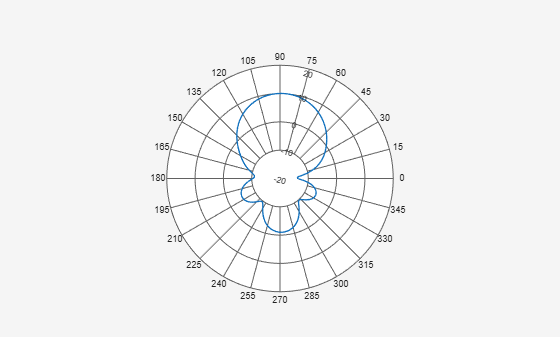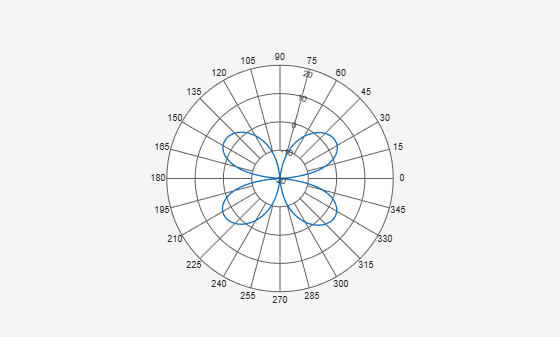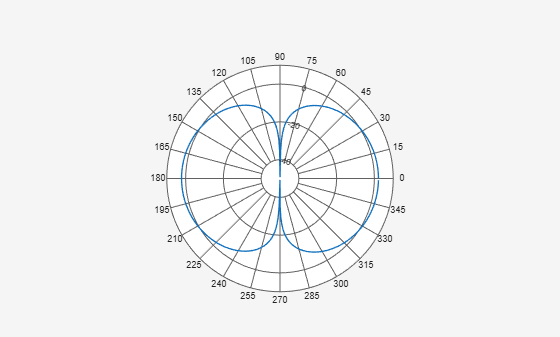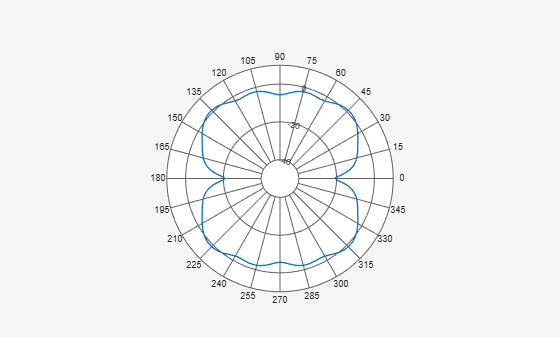# animate

Replace existing data with new data for animation

## Syntax

``animate(p,data)``
``animate(p,angle,magnitude)``

## Description

example

````animate(p,data)` removes all the current data from polar plot, `p` and adds new data, based on real amplitude values, `data`. ```

example

````animate(p,angle,magnitude)` removes all the current data polar plot, `p` and adds new data sets of angle vectors and corresponding magnitude matrices. ```

## Input Arguments

expand all

Polar plot, specified as a scalar handle.

Antenna or array data, specified as one of the following:

• A real length-M vector, where M contains the magnitude values with angles assumed to be $\frac{\left(0:M-1\right)}{M}×{360}^{\circ }$ degrees.

• A real M-by-N matrix, where M contains the magnitude values and N contains the independent data sets. Each column in the matrix has angles taken from the vector $\frac{\left(0:M-1\right)}{M}×{360}^{\circ }$ degrees. The set of each angle can vary for each column.

• A real N-D array, where N is the number of dimensions. Arrays with dimensions `2` and greater are independent data sets.

• A complex vector or matrix, where `data` contains Cartesian coordinates ((x,y) of each point. x contains the real part of `data` and y contains the imaginary part of `data`.

When data is in a logarithmic form such as dB, magnitude values can be negative. In this case,`polarpattern` plots the lowest magnitude values at the origin of the polar plot and highest magnitude values at the maximum radius.

Set of angles, specified as a vector in degrees.

Set of magnitude values, specified as a vector or a matrix. For a matrix of magnitude values, each column is an independent set of magnitude values and corresponds to the same set of angles.

## Examples

expand all

Create a helix antenna that has a 28 mm radius, a 1.2 mm width, and 4 turns. Plot the directivity of the antenna at 1.8 GHz.

```hx = helix('Radius',28e-3,'Width',1.2e-3,'Turns',4); H = pattern(hx, 1.8e9,0,0:1:360); P = polarpattern(H);```Create a dipole antenna and calculate its directivity at 270 MHz.

```d = dipole; D = pattern(d,270e6,0,0:1:360);```

Replace the existing polar plot of the helix antenna with the directivity of the dipole using the `animate` method.

`animate(P,D);`Create a default dipole antenna and plot the polar pattern of its directivity at 1 GHz.

```d = dipole; D = pattern(d,75e6,0,0:1:360); P = polarpattern(D);```Create a default cavity antenna. Calculate the directivity of the antenna and write the data to `cavity.pln` using the `msiwrite` function.

```c = cavity; msiwrite(c,2.8e9,'cavity','Name','Cavity Antenna Specifications');```

Read the cavity specifications file into `Horizontal`, `Vertical` and `Optional` structures using the `msiread` function.

`[Horizontal,Vertical,optional]= msiread('cavity.pln')`
```Horizontal = struct with fields: PhysicalQuantity: 'Gain' Magnitude: [360x1 double] Units: 'dBi' Azimuth: [360x1 double] Elevation: 0 Frequency: 2.8000e+09 Slice: 'Elevation' ```
```Vertical = struct with fields: PhysicalQuantity: 'Gain' Magnitude: [360x1 double] Units: 'dBi' Azimuth: 0 Elevation: [360x1 double] Frequency: 2.8000e+09 Slice: 'Azimuth' ```
```optional = struct with fields: name: 'Cavity Antenna Specifications' frequency: 2.8000e+09 gain: [1x1 struct] ```

Replace data from the dipole antenna with data from cavity antenna.

`animate(P,Horizontal.Azimuth,Horizontal.Magnitude);`## Version History

Introduced in R2016a